• 【写博客常用】Latex参考文献管理：Bibtex教程 Latex参考文献管理：Bibtex教程 这篇文章一直到这儿都是对的 因为有些文章自带这个包，其实没有必要引用，之后可能会出现报错，报错的那一行看看有没有类似&的...
【写博客常用】Latex参考文献管理：Bibtex教程
Latex参考文献管理：Bibtex教程
这篇文章一直到这儿都是对的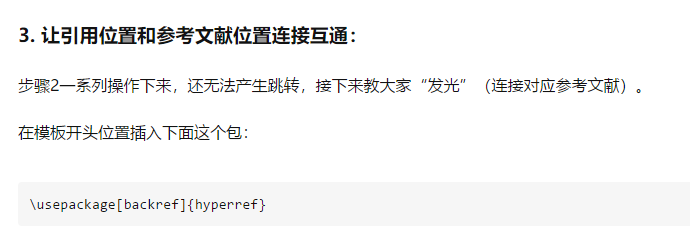因为有些文章自带这个包，其实没有必要引用，之后可能会出现报错，报错的那一行看看有没有类似&的字符，在前面加个\就可


展开全文• ## latex教程详细笔记

万次阅读 多人点赞 2018-05-07 20:41:11
本文的主要参考文献是ta 1.字体大小 options—options interface—Font schemes—Font双击从右边找到FONT_SIZE将10改为自己想要的，如14—-保存—右键单击左边之前的Font—-Load Script—结束 2.分段机制 ...
本文持续更新…
目录本文持续更新...0 包文件1.字体1.1 字体大小1.2 字体加粗1.3 字体显示形式1.3.1 删除字体1.3.2 下标线1.3.3 其他形式2.分段机制3.空格4.自动补全5.数学模式与文本模式5.1 行内公式和行间公式5.2 数学公式编号手动编号自动编号为知笔记Latex马克飞象5.3 数学公式对齐5.4 公式之间空格6.输入函数7.矩阵和大括号8.分段函数9.加载宏包doc文档10.输入中文以及宏包安装11.插入图片12.表格12.1普通表格调整表格和标题/标注的距离多行12.2合并行列 1,合并行 2,合并列 3,行列都存在12.3表格下标注释和字体大小设置设置表格（字体）大小彩色表格和字体彩色表格彩色字体12.4 图片表格并列12.5 图片和图片并列12.5 图片上下并排12.6 表格和表格并列两个表格并列多个表格并列12.7 更多关于表格的举例12.8 空行13.交叉引用（corss-reference）14.文章布局（1）页面大小（2）页眉页脚（3）列举（4）参考文献A.手动输入B.使用Bibtex15.超链接16.插入代码17.目录生成18. 段落空行, 换行和首部缩进
本文是笔者初学WinEdt用以编辑Latex的笔记，只涉及一些简单问题，详细请参考百度文库（点点这几个字看看~~）
本文的主要参考文献是ta
0 包文件
这里主要给出本文所有的头文件，即需要使用的包。在运行本文中的事例之前在开头复制以下代码
% !Mode::"TeX:UTF-8"
%\documentclass[prd,aps,onecolumn,preprintnumbers,amsmath,amssymb,superscriptaddress]{article}
\documentclass{article}
%\usepackage[UTF8]{ctex}
\usepackage{listings}
\usepackage{amsthm}
\usepackage{amsmath}
\usepackage{amssymb}
\usepackage{subfigure}
\usepackage{graphicx}
\usepackage{hyperref}
\usepackage[table]{xcolor}
\usepackage{fancyhdr}
\usepackage{footnote}
\usepackage{lastpage}
%\usepackage{pythonhighlight}
\usepackage{indentfirst}
\usepackage{multirow}
\usepackage{authblk}
\usepackage{comment}
\usepackage{url}
\usepackage{float}
\usepackage{cite}
\usepackage{caption}
%\usepackage{graphix}
\setlength{\parindent}{1em}
%\pagestyle{fancy}
%设置页面大小
%\chead{LSPE}
%\rhead{\thesection}
%\cfoot{Page \thepage of \pageref{LastPage}}
\usepackage[top=2.54cm, bottom=2.54cm, left=3.18cm,right=3.18cm]{geometry}

1.字体
1.1 字体大小
1 设置latex/winedt代码中字体大小
options—options interface—Font schemes—Font双击从右边找到FONT_SIZE将10改为自己想要的，如14----保存—右键单击左边之前的Font----Load Script—结束
2 设置文章字体大小

\tiny
\scriptsize
\footnotesize
\small
\normalsize
\large
\Large
\LARGE
\huge
\Huge

比如：
{\small Hello word}

花括号框住的地方都变小
1.2 字体加粗
文本加粗
\textbf{这里放文本}

既要加粗又要变小：（为毛感觉有点邪恶~~ 2333333  认真脸）
{\textbf{\small Hello word}}

数学符号加粗
$\boldsymbol 直接接数学符号$

1.3 字体显示形式
1.3.1 删除字体
\usepackage{cancel}
\cancel{abc}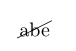1.3.2 下标线
这里解决的问题是下标线导致的问题，如
f_x

结果为：$f_x$
而我想要的结果是下划线一起输出，我们需要一个转义字符\即可，
f\_x

$f\_x$
1.3.3 其他形式
\cancel{abe}
\textbar{abc}
\textcircled{acbd}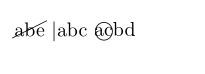更多形式可以在\text…自动补全查看
2.分段机制
1, 在原码中空一行，则生成的pdf会换行
2, 在文后使用双斜杠\
3, 使用命令
\newparas

举例：
Life is too short so that I use python\\
me too.

Life is too short so that I use python

me too.

Life is too short so that I use python
\newparas
me too.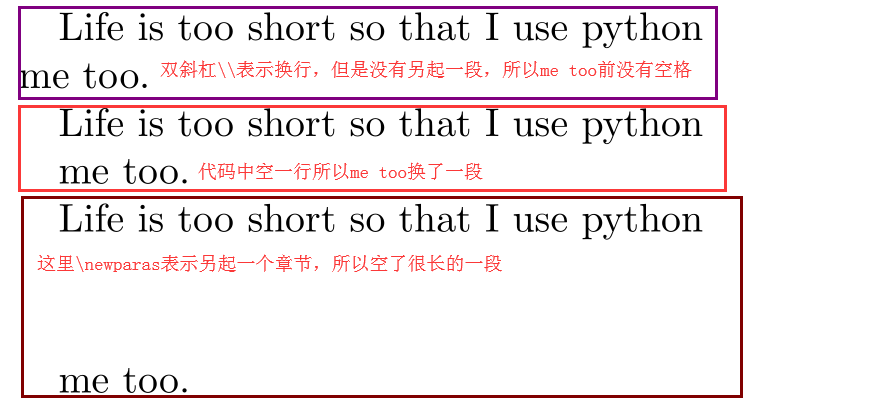3.空格
参考自：seaskying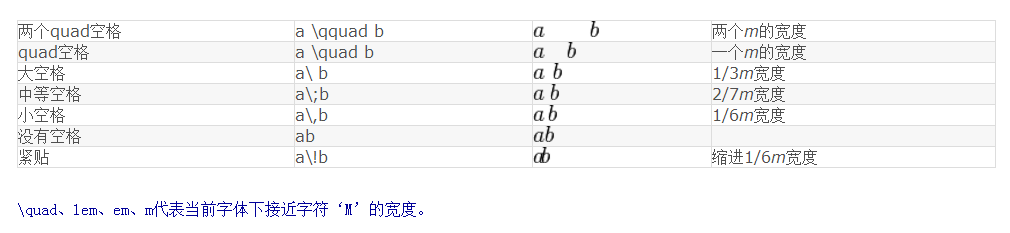当然直接空一格也是可以得到一个空格的，如下：
  $a$, $b$, $c$.

$a,\ b,\ c.$

The first three letter are a, b, c.

结果为：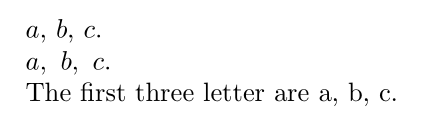所以如果要空格直接按空格键即可，即上面代码的1和3方式
4.自动补全
快捷键：Ctrl+Enter
5.数学模式与文本模式
5.1 行内公式和行间公式
引入数学宏包{amssyb},American mathematics society symbol.例句如下
% !Mode::"TeX:UTF-8"
\documentclass{article}
\usepackage{amssymb}
\begin{document}
\section{symbol}
1+1=2, $$1+1=2$$, $I know 1+1=2,$

This is in text mode, $This is in math mode,$ $This\ is\ in\ math\ in\ mode.$

\end{document}

结果如下：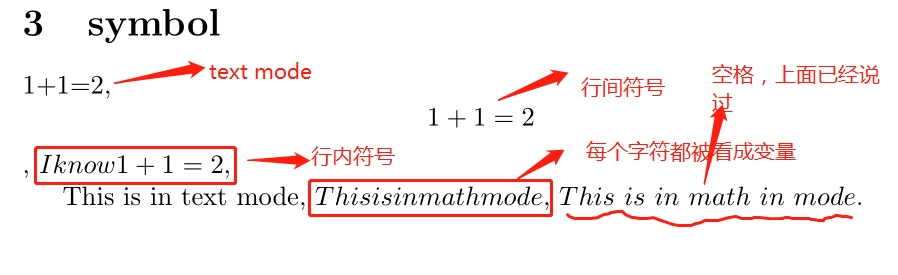对行内公式和行间公式总结如下：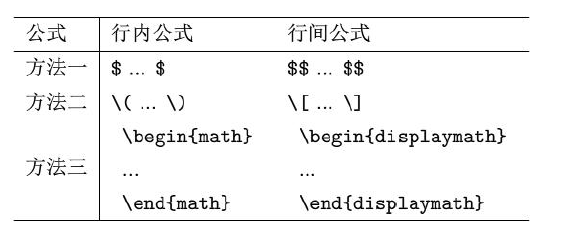上面的方法是无法给公式编号的
5.2 数学公式编号
手动编号
通过采用\tag来编号，当引用公式时手动输入。缺点是当更改公式里的编号时引用中的编号不能自动更改，其二，不同通过点击引用的编号回到公式
$$y=1+e^x \tag{1.1}$$
我们今天要讨论这个方程$(1.1)$

$y=1+e^x \tag{1.1}$
我们今天要讨论这个方程$(1.1)$
这个好处是在处理文章比较长，有很多节，如写书等时，可以手动写(1.1),(2.1)(2.1.1)等公式，而自动则是(1)(2)这样排序，没有分小节。
自动编号
建立公式环境，如下：
\begin{equation}\label{加入标记}
xxx
\end{equation}

不同的平台渲染不一样，比如为知笔记, latex等可以通过以下方法渲染自动编号
为知笔记
$$\begin{equation}\label{my_equ1} a^2+b^2=c^2 \end{equation}$$
We will use equation \eqref{my_equ1}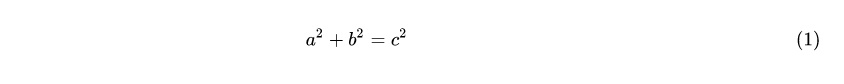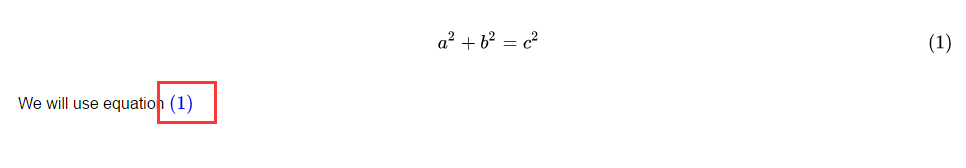Latex
对于latex而言，不需要这样的符号 \begin{equation}\label{time_pixel} t_\text{pix}=\frac{\Delta T}{N_\text{pix}}\times N_\text{det}. \end{equation} Substituting \autoref{time_pixel}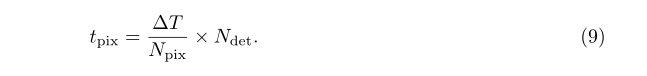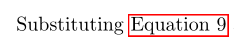马克飞象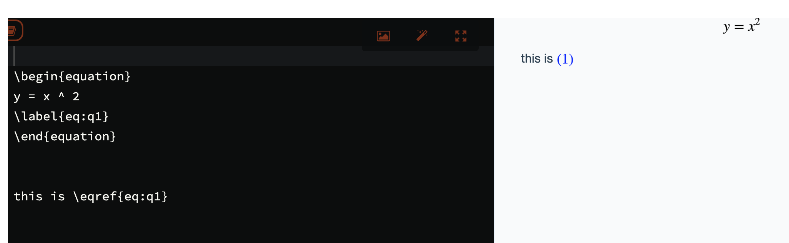（马克飞象有时候渲染会失败，跟官方人员问过，给的办法是：失败的时候重新打开就可以看到自动编号，但是遗憾的是，当你尝试改动时又会出错，因此官方建议先编写完，再渲染，这样就不会去动它了。。。。呵呵哒。） 5.3 数学公式对齐 采用{aligned}如下： \begin{equation}\label{information gain} \begin{aligned} Gain(X,Y)&=H(Y)-H(Y|X)\\ &=H(X)-H(X|Y)\\ &=H(X)+H(Y)-H(X,Y) \end{aligned} \end{equation}  结果为：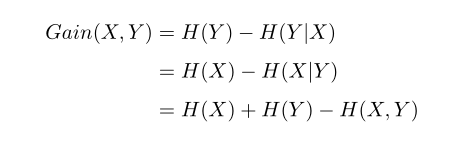\begin{equation}\label{lagrangian} \begin{aligned} w_{ij}&=\frac{1}{(N_i+R_i)(N_j+R_j)\frac{1}{2}(1+\delta_{ij})}\\ Var[\mathbb{\hat{C}}_\ell]&=\frac{2}{2\ell+1}\left(\sum_{i,j,j\ge i}\frac{1}{(N_i+R_i)(N_j+R_j)\frac{1}{2}(1+\delta_{ij})}\right)^{-1} \end{aligned} \end{equation}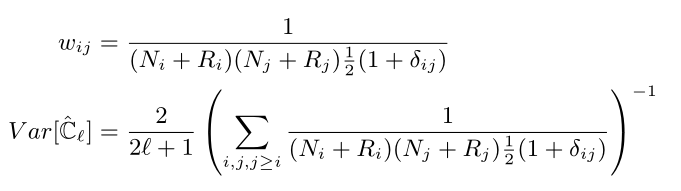更新 对上面的多行对其进行更新补充(2021.3.24) 上面的多行对齐使用的是aligned不是align或者align*，后者会存在一定问题，即equation已经开启了一个数学模式，align又开启一个，这将会导致数学模式的嵌套，但aligned是没问题的。 现在将equation和align分开，使用的包是amsmath \usepackage{amsmath}  1）对齐且每个公式后都有编号 \begin{align} y&=\cos x\\ z&=\sin x \end{align}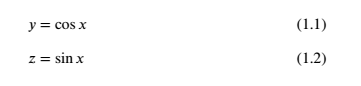2）对齐但没有编号，对align加一个星号即可 \begin{align*} y&=\cos x\\ z&=\sin x \end{align*}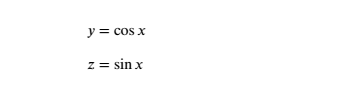3）对齐只有一个编号 使用split将公式分行，并会在中间位置编号。 \begin{equation} \begin{split} y&=\cos x\\ z&=\sin x \end{split} \end{equation}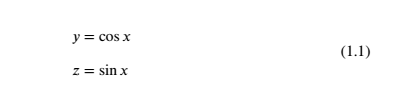为知笔记的对齐 跟上面一样，但是为知笔记这样的缺点是，不能再使用\eqref{label}的方法引用了，所以也是一大缺点 \begin{equation}\label{cos_age} \begin{aligned} t_0&=\int \ dt\\\ &=\frac{1}{H_0}\int_{0}^{1}\frac{da}{a\sqrt{\Omega_{\Lambda0}+\Omega_{k0}(a_0/a)^2+\Omega_{m0}(a_0/a)^3+\Omega_{r0}(a_0/a)^4}} \end{aligned} \end{equation}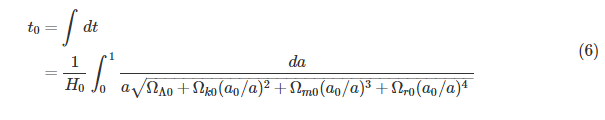5.4 公式之间空格 没有空格 \begin{equation}\label{add_prior} \frac{\sigma(A_s^p)}{A_s^p}=0.17, \sigma(\beta_s)=0.17, \frac{\sigma(A_d^p)}{A_d^p}=0.015, \sigma(\beta_d)=0.02 \end{equation}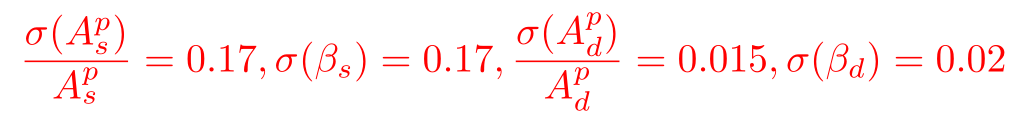发现上面的公式之间挨得太近了，想要空格，可以使用第3部分提到的空格方法，将上面的代码增加\quad： \begin{equation}\label{add_prior} \frac{\sigma(A_s^p)}{A_s^p}=0.17,\quad \sigma(\beta_s)=0.17,\quad \frac{\sigma(A_d^p)}{A_d^p}=0.015,\quad \sigma(\beta_d)=0.02 \end{equation}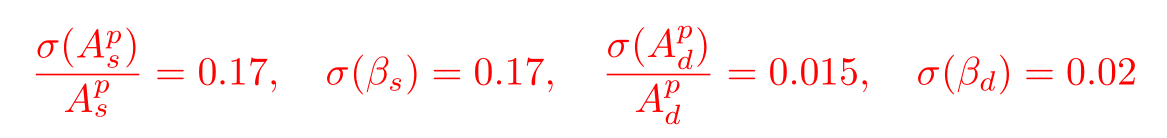6.输入函数 输入函数前面加斜杠"" 如： \frac{10}{11}, \sqrt{x^2+1}, \ldots, \sin x, \cos x.  结果如下：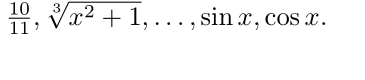可以看到，虽然在上面代码每个逗号之后都输入了空格，但得到的pdf文档并没有逗号，而英文中是习惯在逗号，句号等符号之后加空格的，这个时候可以将上面代码改为： \frac{10}{11}, \sqrt{x^2+1}, \ldots, \sin x, \cos x.  即每个函数单独用美元符号分开，并在两者之间加符号。  \sum_{n=1}^{\infty}\sum_{n=1}^{\infty}\lim_{n \to \infty}(1+\frac{1}{n})^n = e,\int_{-\infty}^{+\infty}\frac{\sin x}{x} \mathrm{d}x=I$$\alpha,\beta,\gamma,\delta\epsilon\varepsilon\xi\pi\rho\sigma\eta\theta\phi\varphi\omega \Gamma\Phi |A|,\|a\|,\vec{a},\overleftarrow{AB},\tilde{x},\widetilde{xyz},sin,\mathrm{sin} \mathbb{AB},\mathbf{abAB}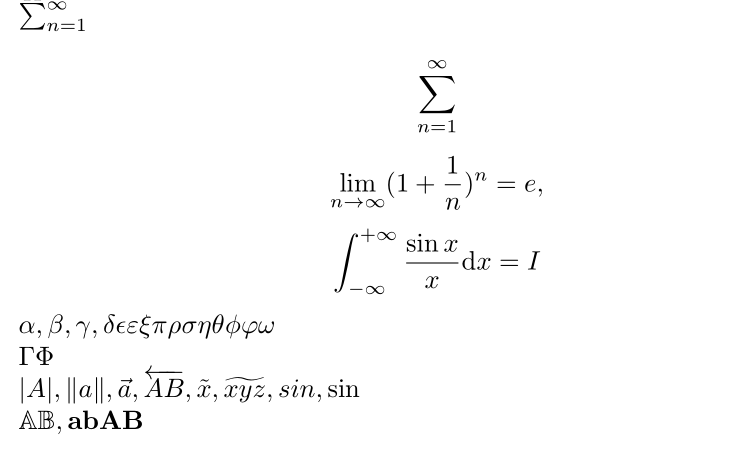（1）第一行是行内公式和第二行的行间公式是不一样的，这是出于文本美观的目的。 （2）\mathrm{d}那儿是为了和x不同，因为d是直立的而不是变量，而x是变量，这里mathrm=math roman （3）剩下的看到gamma等希腊字母第一个字母大写则表示输出的文本是大写，这里值得注意的是：alpha,beta,varepsilon等是没有大写形式的，因为其大写形式就是ABE，不能输入\Alpha,\Beta… （4）mathbb=math blackboard, mathbf=math boldface 实际上第行中的括号应该是大括号而不是小括号，细节见下节。 7.矩阵和大括号 先看矩阵  \begin{equation} \left( \begin{array}{ccc} a_{11}& a_{12} & a_{13} \\ a_{21} & a_{22} & a_{23} \\ a_{31} & a_{32} & a_{33} \end{array} \right) \end{equation}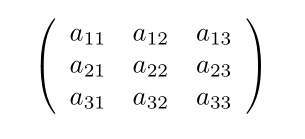如果需要写 “函数=矩阵” 的形式，只需要在\left前加入即可，如： \begin{equation} f= \left( \begin{array}{ccc} a_{11}& a_{12} & a_{13} \\ a_{21} & a_{22} & a_{23} \\ a_{31} & a_{32} & a_{33} \end{array} \right) \end{equation}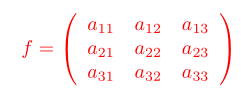（这里不要纠结颜色，因为我都改为红色了） （1）以上begin{equation}开始了一个公式环境，在这个环境中可以直接输入变量、函数等而不需要加符号，上面等同于 $$ \left(
\begin{array}{ccc}
a_{11}& a_{12} & a_{13} \\
a_{21} & a_{22} & a_{23} \\
a_{31} & a_{32} & a_{33}
\end{array}
\right)$$ （2）\left( and \right) are left bracket and right bracket. 能够通过括号内的内容自动改变括号大小。所以上面6中的第三行应该改为： $$\lim_{n \to +\infty}\left(1+\frac{1}{n}\right)^n=e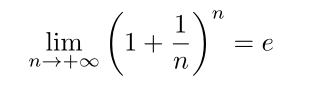（3）符号&是用来分隔列的，同一行不同列之间的元素用&分隔开
（4）｛ccc｝是array中必选参数，为center,center,center,表示三列都居中，
（5）\是强制换行符号
\begin{equation}\label{Cx1}
C_{X\ell}^{R_1}=\frac{A^2}{16\pi}\sum_{\ell_1}(2\ell_1+1)C_{X\ell_1}^f\sum_{\ell_2}(2\ell_2+1)C_{\ell_2}^\beta\left(
\begin{array}{ccc}
\ell&\ell_1&\ell_2\\
2&-2&0
\end{array}
\right)
\end{equation}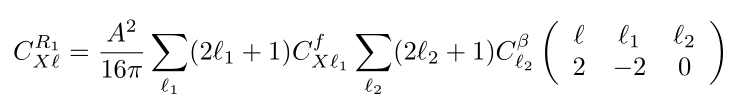\begin{equation}\label{Cx1}
C_{X\ell}^{R_1}=\frac{A^2}{16\pi}\sum_{\ell_1}(2\ell_1+1)C_{X\ell_1}^f\sum_{\ell_2}(2\ell_2+1)C_{\ell_2}^\beta\left(
\begin{array}{ccc}
\ell&\ell_1&\ell_2\\
2&-2&0
\end{array}
\right)
\left(
\begin{array}{ccc}
\ell&\ell_1&\ell_2\\
2&-2&0
\end{array}
\right)
\end{equation}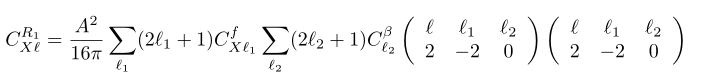8.分段函数
分段函数是在矩阵的基础之上的，比如如下的分段函数
  \begin{equation}
f(x)=
\left\{
\begin{array}{cc}
\int \mathrm{d}^3p\int_{0}^{\infty}\mathrm{d}x x^2 \frac{\delta(E-\sqrt{p^2+m^2})}{2E} , & x \geq 0 \\
0,& x < 0
\end{array}
\right.
\end{equation}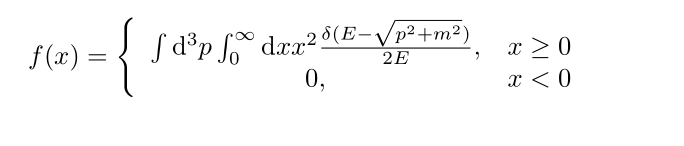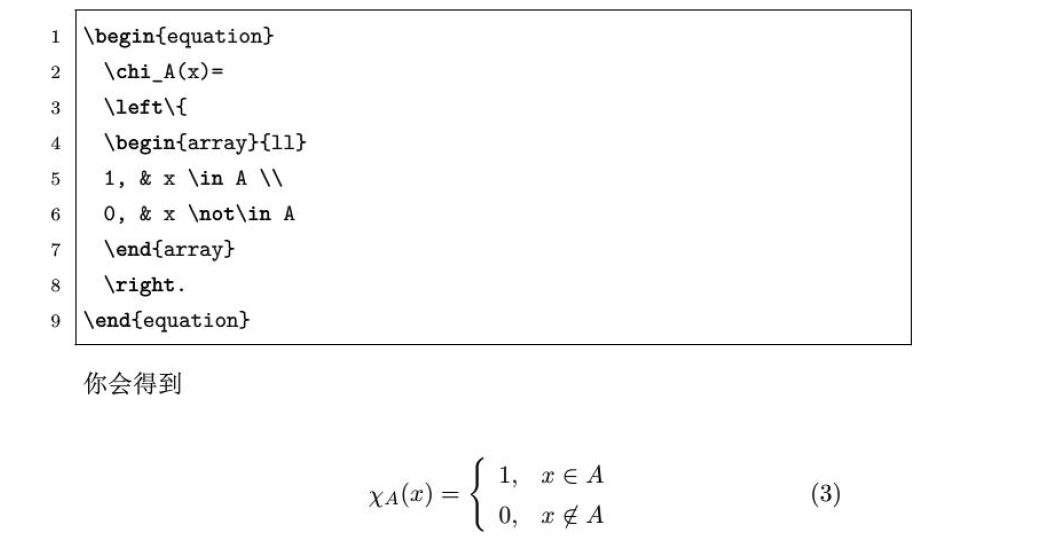（1）不需要右括号
（2）&的作用是使得列对齐
9.加载宏包doc文档
当我们使用一个宏包比如amsmath，想要得到说明文时可以如下操作：
在控制台上点击终端cmd窗口，输入tex xxx(xxx是宏包名，如amsmath)回车即可。
终端按钮如下图：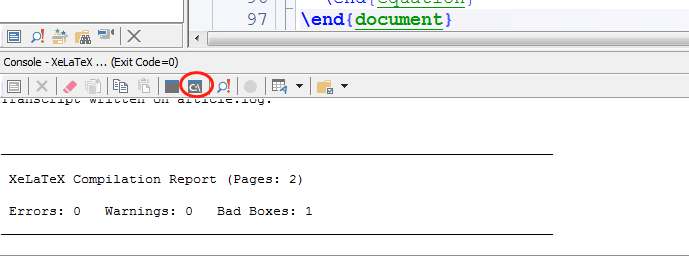10.输入中文以及宏包安装
输入中文需要用到ctex包，这是专门针对中文而设计的包，使用时在前面将
\document{article}

改为
\document[UTF8]{ctexart}

即可,（有的不需要加方括号中的内容），或者\document{article}不变，在其下加入一个宏包为
\usepackage[UTF8]{ctex}

此外，中文包还可以使用
\usepackage{CJK}

当然，前提是已经安装ctex宏包，通常情况都会自动安装好，如果没有安装可以如下（笔者用的是Tex live）
开始—tex live—tex live manager—找到ctex—点击安装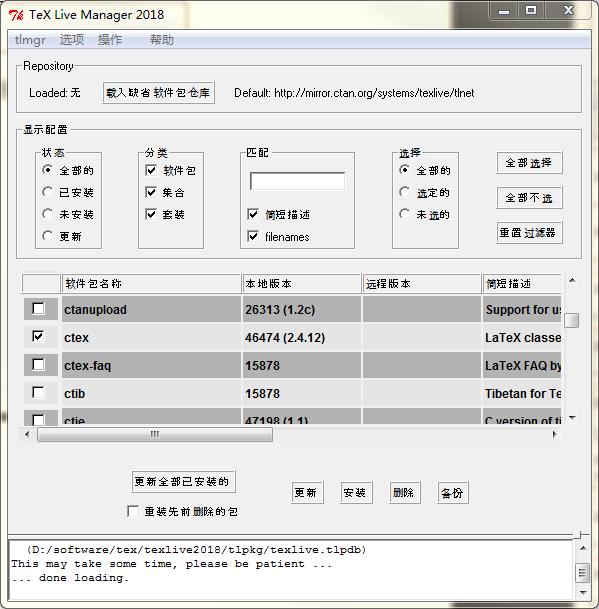值得注意的是：宏包已经都不需要网上下载安装，而是直接从库上安装缺少的宏包，方法就是上面的方法。
11.插入图片
插入图片可以引用命令：
\ref{fig} or \autoref{fig}

前者只给图片序号，而后者为Figure + 序号
插入图片需要加载宏包
\usepackage{graphicx}

或
\usepackage{graphics}

建议前者。
插入图片的环境为：
  \begin{figure}[ht]
\centering
\includegraphics[width=10cm]{hh}
\caption{这个是图片}\label{bbb}
\end{figure}

解释：
（1）第一句begin{figure}代表开始插入图片，后面的[ht]是可选参数，表示here, top。如果去掉[ht]表示默认的htbp,分别表示“这里，顶部，底部，换一页”（here, top, bottom, page）
（2）第二句是图片居中，否则自动靠左
（3）[width=10cm] (有些people不需要加cm，是已经设置单位是cm了，还可以有in英尺)，可选的参数还有如下（可参考DreamSeeking的文献）：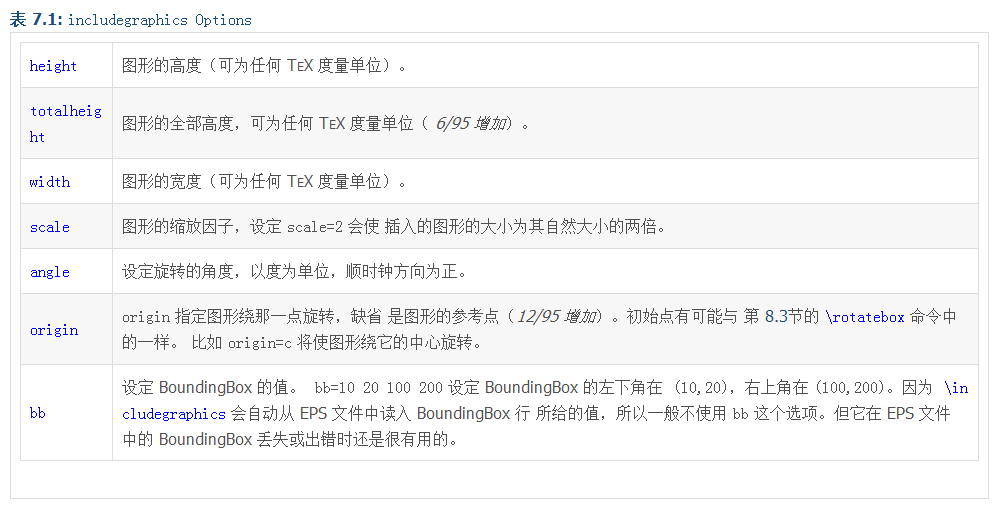第三行最后的｛hh｝表示插入的图片的名字，因此插入的图片一定要放在工作目录下，并命名为hh.xxx（xxx表示后缀，如png,jpg,eps等）
当然也有不在工作目录下的方法，这个google一下by yourself.在此不累赘。（好吧，让我再告诉你好了，其实就是使用绝对路径
\includegraphics[width=10cm]{D:/software/tex/hh.png}

当然，.png后缀可以不写）注意，绝对路径中是用的左斜杠“/”而不是右斜杠。
此外如果图片比较多，最好的方法是在当前工作路径下单独建一个文件夹，命名为fig.然后将图片都放进去，此后要使用里面的图片时只需要使用路径加载即可，这是相对路径方法，如下：
\includegraphics[width=10cm]{fig/hh}

（4）caption｛这个是图片｝表示对图片的描述，将出现在图片的底下。label{bbb}表示对这张图片的标签，相当于给定了这张图片的名字。图片较多时，建议使用容易看懂的名字，如label{fig.sub.1}。这个label的作用是方便以后引用这个图时只需要用其标签就可以，比如
see,figure\ref{fig.sub.1} or \autoref{fig.sub.1}

结果就是：see,figure x（x表示刚刚插入的图的序号，如图3）
（5）这样插入的图片是一个浮动图，也就是latex自动找到最好的位置来放置此图，而不是你代码设置的地方，想要得到不浮动的图可以将
\includegraphics[width=10cm]{fig/hh}

这句单独提取出来放置想要放的代码中即可，但这个时候是不能加
\caption{xxx}

这一句的，也就是没法加入标题。如果要给不浮动的图形加标题，那么可以看看nonfloat 包或者自己google(这回是朕是真的不打算给你找了)。
12.表格
12.1普通表格
表格制作可以参考这个 and 这个（点一下试试），后者是英文。
简单说明：
表格制作可以使用tabular，简单一个图如下：
  \begin{tabular}{|c|c|}
\hline
% after \\: \hline or \cline{col1-col2} \cline{col3-col4} ...
Name & score \\
\hline
小明 & 50 \\
小华 & 45 \\
\hline
\end{tabular}

结果如下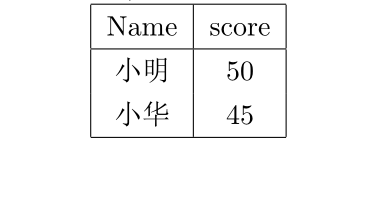讲解：
（1）第一句是tabular表格的开始，参数{|c|c|}中的第一个|表示表格中的左边竖，第二、三个分别是中间和右边的竖。而c表示表格中的字体居中，l(left)和r(right)表示表格中字体靠左和靠右。可以尝试将｛|c|c|｝改为{c|c}即不要两边的竖线。
（2）\hline（horizontal line）表示画一条水平线
（3）%是注释，\是换行，\hline可以紧接在\后，如下表示：
\\ \hline

会显得更紧凑。
当然，这个表格是不浮动的，和图片一样，如果想加入\caption的话，可以构成浮动的图片（但毕竟可以写标题了呀，想想还是赚的）。这个时候需要用到table环境。如下
  \begin{table}
\centering
\begin{tabular}{|c|c|}
\hline
% after \\: \hline or \cline{col1-col2} \cline{col3-col4} ...
Name & score \\
\hline
小明 & 50 \\
小华 & 45 \\
\hline
\end{tabular}
\caption{这是一个表格}
\end{table}

同样的\centering是表格居中，否则居左。其他都讲过了，很easy.最后可以使用caption宏包，这个需要自行google。
在表格中换行
比如两个表格(table)是要构成一个table的，想统一做一个说明，而不是每个table单独说明，此时需要将两个表格分开，这个时候可以用命令：
\vspace{0.35cm}   #表示上下空0.35cm的空间

比如
\begin{table}
\centering
%\footnotesize
Case 0: 95+150

\begin{tabular}{|c|c|c|c|c|c|}
\hline
$r$ & $\sigma_r(\sigma_F=1)$ & $\sigma_r(\sigma_F=0.1)$ & $\sigma_r(\sigma_F=0.01)$ & $N_{95}$ & $N_{150}$\\
\hline
0.01 & 0.01094 & 0.00228 & 0.00106 & 8701 & 1299 \\
0 & 0.01084 & 0.00216 & 0.00091 & 8701 & 1299 \\
\hline
\end{tabular}
\vspace{0.35cm}    #空0.35cm

Case 1: 41+95+150

\begin{tabular}{|c|c|c|c|c|c|}
\hline
$r$ & $\sigma_r(\sigma_F=1)$ & $\sigma_r(\sigma_F=0.1)$ & $\sigma_r(\sigma_F=0.01)$ & $N_{95}$ & $N_{150}$\\
\hline
0.01 & 0.00787 & 0.00203 & 0.00106 & 8701 & 1299 \\
0 & 0.01084 & 0.00216 & 0.00091 & 8701 & 1299 \\
\hline
\end{tabular}
\caption{table 1}\label{anser1}
\end{table}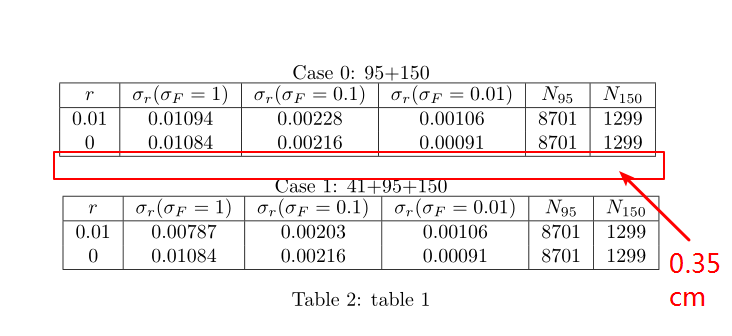如果要改变字体大小，只要在第二行即\centering下加入改变字体大小的代码即可，（如我已经注释掉的\footnotesize），更多字体大小的代码见第一部分。
调整表格和标题/标注的距离
可以使用以下两个命令，关于调节距离多少，可以尝试调节里面的数字
\setlength{\abovecaptionskip}{0.cm}

\setlength{\belowcaptionskip}{-0.9cm}

多行
现在要将上面的tabe2设置为如下形式，即对应r=0.01有多个$\sigma_F$可选。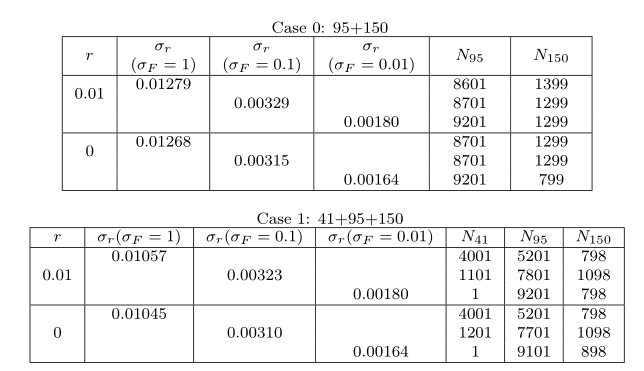代码如下：
\begin{table}
\centering
\footnotesize
Case 0: 95+150

\begin{tabular}{|c|c|c|c|c|c|c|}
\hline
\multirow{2}{0.25cm}{\centering $r$} & \multirow{2}{1.2cm}{\centering $\sigma_r$\\$(\sigma_F=1)$} & \multirow{2}{1.4cm}{\centering $\sigma_r$\\$(\sigma_F=0.1)$} & \multirow{2}{1.6cm}{\centering $\sigma_r$\\$(\sigma_F=0.01)$} & \multirow{2}{1cm}{\centering $N_{95}$} & \multirow{2}{1cm}{\centering $N_{150}$}\\
& & & & & \\
\hline
\multirow{2}*{0.01} & 0.01279 & & & 8601 & 1399 \\
& & 0.00329 & & 8701 & 1299 \\
& & & 0.00180 & 9201 & 1299 \\
\hline
\multirow{2}*{0} & 0.01268 & & & 8701 & 1299 \\
& & 0.00315 & &8701 & 1299\\
& & & 0.00164 & 9201 & 799 \\
\hline
\end{tabular}
\vspace{0.35cm}

Case 1: 41+95+150

\begin{tabular}{|c|c|c|c|c|c|c|}
\hline
$r$ & $\sigma_r(\sigma_F=1)$ & $\sigma_r(\sigma_F=0.1)$ & $\sigma_r(\sigma_F=0.01)$ & $N_{41}$ & $N_{95}$ & $N_{150}$\\
\hline
& 0.01057 &  &  & 4001 & 5201 & 798 \\
0.01 &  & 0.00323 & & 1101 & 7801 & 1098 \\
& & & 0.00180 & 1 & 9201 & 798 \\
\hline
& 0.01045 & & & 4001 & 5201 & 798 \\
0 & & 0.00310 & & 1201 & 7701 & 1098 \\
& & & 0.00164 & 1 & 9101 & 898 \\
\hline
\end{tabular}
\caption{table 1}\label{anser1}
\end{table}

12.2合并行列
1,合并行
使用
\multirow{n}*{text}   #n表示要合并的行数，*是必须的，否则无法编译成功，text是此行中要输入的内容

注意，使用\multirow一定要在导言区，也就是在最开头使用包\usepackage{multirow}
举例如下：
% !Mode::"TeX:UTF-8"
\documentclass{article}
\usepackage[UTF8]{ctex}
\usepackage{listings}
\usepackage{amsthm}
\usepackage{amsmath}
\usepackage{amssymb}
\usepackage{graphicx}
\usepackage{hyperref}
\usepackage[table]{xcolor}
\usepackage{fancyhdr}
\usepackage{lastpage}
\usepackage{pythonhighlight}
\pagestyle{fancy}
\usepackage{enumitem}
\setlist[trivlist]{topsep=\baselineskip}
\usepackage{multirow}

%这里以上都是导言区

\begin{document}
\begin{tabular}{|c|c|c|}
\hline
\multirow{3}*{朕} & 尚书省 & 工部  刑部  兵部  礼部  户部  吏部 \\
\cline{2-3}
& 中书省 & ... \\
\cline{2-3}
& 门下省 & ... \\
\hline
\end{tabular}
\end{document}

得到如下图：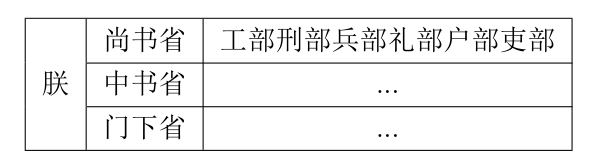上面中 \cline{a-b}表示从第a列画一条到第b列的线，与\hline的区别在于可以指定线的起始位置。
2,合并列
使用
\multicolumn{n}{x}{text}  #n为合并列的数量，x为字体的排版样式，比如r,l,c分别表示右对齐，左对齐，居中，text为内容

例子
\begin{document}
\begin{tabular}{|c|c|}
\hline
\multicolumn{2}{|c|}{成绩} \\
\hline
语文 & 数学 \\
98 & 100 \\
\hline
\end{tabular}
\end{document}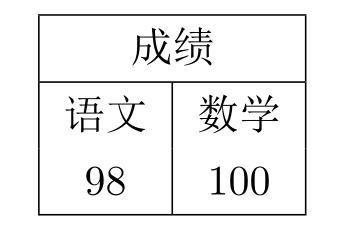3,行列都存在
\begin{document}
\begin{tabular}{|c|r|r|}
\hline
\multirow{2}*{姓名} & \multicolumn{2}{c|}{成绩}  \\
\cline{2-3}
& 语文 & 数学 \\
\hline
小明 & 60 &  55 \\
\hline
\end{tabular}
\end{document}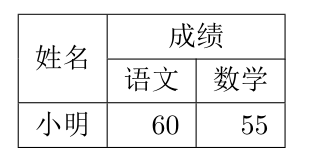12.3表格下标注释和字体大小设置
给定一个表格后，表下可能会有表格的介绍，而介绍的字体通常比文章字体小，可以用
\small{}

  \begin{table}
\centering
\rowcolors[\hline]{2}{orange}{red!30!yellow}
\begin{tabular}{|c|c|}
\rowcolor{red!20}
Name & score \\
小明 & 50 \\
小华 & 45 \\
\hline
\end{tabular}
\caption{\small{This is a simple table for testing}}
\end{table}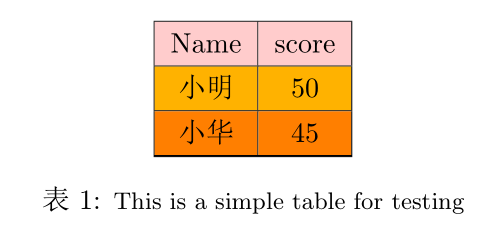设置表格（字体）大小
同样，可以通过\small来修改，但是放置的位置和上面不同，而是放在\begin{table}之后，如：
  \begin{table}
\centering
\small    #改变表格字体大小，同时表格大小也会变
\rowcolors[\hline]{2}{orange}{red!30!yellow}
\begin{tabular}{|c|c|}
\rowcolor{red!20}
Name & score \\
小明 & 50 \\
小华 & 45 \\
\hline
\end{tabular}
\caption{\small{This is a simple table for testing}}
\end{table}

此外还有

\tiny
\scriptsize
\footnotesize
\small
\normalsize
\large
\Large
\LARGE
\huge
\Huge

彩色表格和字体
彩色表格
彩色表格需要用到宏包
\usepackage{xcolor}

没有需要按照上面的方法先安装。因为是针对表格，所以需要支持表格，使用时要如下：
\usepackage[table]{xcolor}

上面的例子可以加入彩色如下：
...
\usepackage[table]{xcolor}
...
...
\begin{table}
\centering
\rowcolors[\hline]{2}{orange}{red!30!yellow}
\begin{tabular}{|c|c|}
\rowcolor{red!20}
Name & score \\
小明 & 50 \\
小华 & 45 \\
\hline
\end{tabular}
\caption{这是一个表格}
\end{table}

结果为：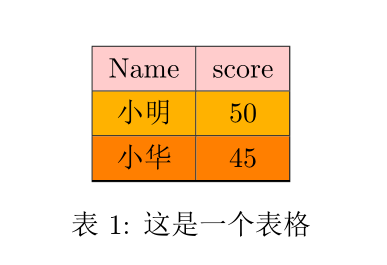说明：
（1）第一行使用宏包xcolor，并支持table
（2）
  \rowcolors[\hline]{2}{orange}{red!30!yellow}

这里是表示行颜色的设置，\hline表示每行都加横线，后面表示从第二行开始奇数行用orange颜色，偶数行用30%红色和30%黄色的混合，也可以写成{red!30yellow!30}
（3）因为我们是从第二行开始加颜色，所以第一行要单独加颜色，如下
\rowcolor{red!20}

如果没有则是白色。如果想要单独每行自行设置，也可以如下单独每行设置颜色
  \begin{table}
\centering
\begin{tabular}{|c|c|}
\hline
\rowcolor{red!20}
Name & score \\
\hline
\rowcolor{yellow}
小明 & 50 \\
\hline \rowcolor{orange}
小华 & 45 \\
\hline
\end{tabular}
\caption{这是一个表格}
\end{table}

彩色字体
同样是xcolor包
{\color{violet}{哇，这是我的第一个 \LaTeX{} Article!}}这一行是通过一个大花括号括起来的（即最左和最后的括号），如果没有则整篇文章都是这种颜色的字体，括起来后就只有这一句话是这个颜色。
更多颜色设置见官网 and it’s pdf article
12.4 图片表格并列
可以参考 https://tex.stackexchange.com/questions/377998/how-to-make-table-and-figure-side-by-side-appear-at-the-same-height
\begin{figure}   %开始图片（图片和表格合成一个大的图片，这里指的是整体的大图片）
\begin{minipage}[b]{.5\linewidth}     %开辟一个小页面，即用来放第一个图片
\centering
\includegraphics[width=6cm]{Figure_1.eps}
\caption{Image}
\end{minipage}%
\begin{minipage}[b]{.5\linewidth}    %开辟第二个小页面，用来放表格
\centering
\begin{tabular}{|c|c|}
\hline
aa & bb \\ \hline
cc & dd \\ \hline
\end{tabular}
\captionof{table}{Table}
\end{minipage}
%\caption{pic and table side by side}
\end{figure}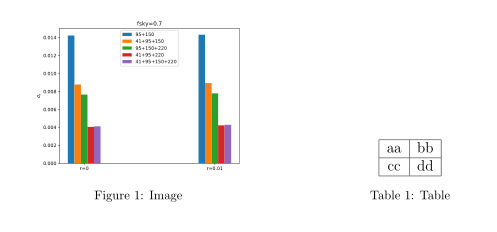注意上面表格和图片合成了一个大的图片,同时可以对上面两个一起写注释，即将上面的
%\caption{pic and table side by side}

去掉注释即可
12.5 图片和图片并列
受上面的启发，我们可以将两张图片并列
\begin{document}

\begin{figure}
\begin{minipage}[b]{.5\linewidth}
\centering
\includegraphics[width=6cm]{large_full.eps}
\caption{Image}
\end{minipage}%
\begin{minipage}[b]{.5\linewidth}
\centering
\includegraphics[width=6cm]{large_part}
\caption{image2}
\end{minipage}
\end{figure}

\end{document}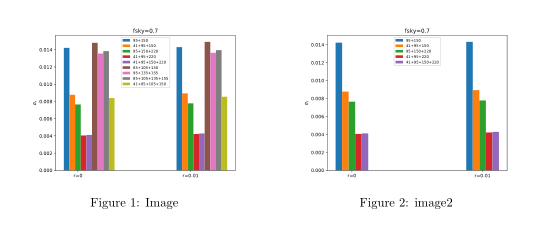除此之外，也可以使用另一种方法，如下：
\begin{figure}[htb]
\centering
\subfigure[]{\includegraphics[width=6.5cm]{large_part.eps}}  %[ ]里面不填就会默认为(a)
\quad
\subfigure[]{\includegraphics[width=6.5cm]{large_full.eps}}    %[ ]里面不填默认为(b)
\caption{\small{The changing of uncertainty $\sigma_r$ on tensor-to-scalar ratio $r$ with the distribution of detectors on focal plane for different frequency combinations with $r$=0.01 and $\sigma_F$=0.1. Contour (a), (b) and (c) correspond to the case 0, case 1 and case 1*, respectively. }}\label{large_fig1}
\end{figure}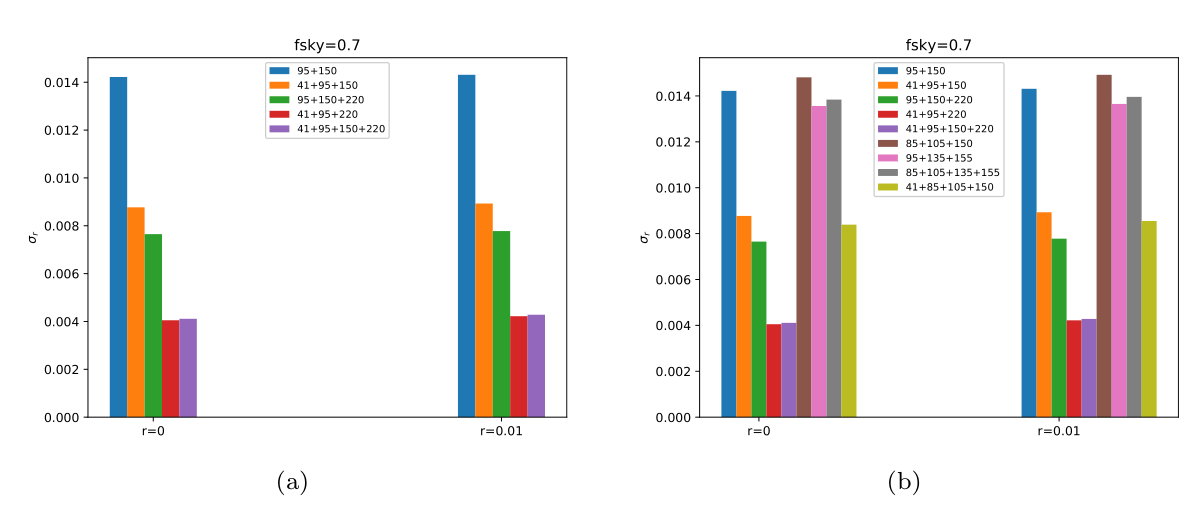更新。。。。
跟第一个方法有点像：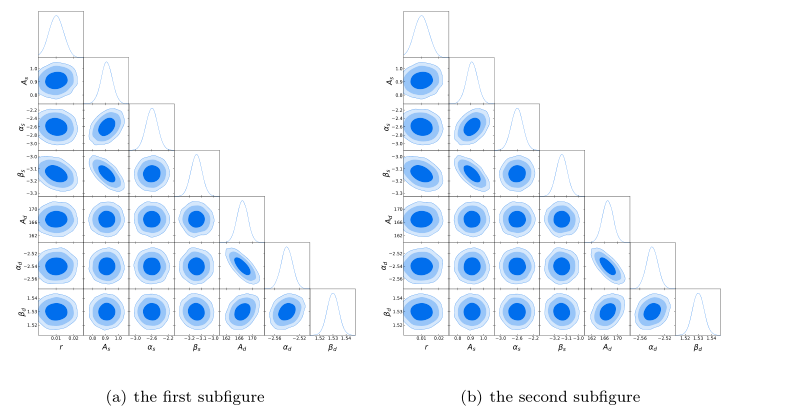\begin{figure}[htb]
\centering
\subfigure[the first subfigure]{
\begin{minipage}[c]{0.4\textwidth}
\includegraphics[width=1\textwidth]{41_95_220_large_corre2.eps} \\
\end{minipage}
}
\subfigure[the second subfigure]{
\begin{minipage}[c]{0.4\textwidth}
\includegraphics[width=1\textwidth]{41_95_220_large_corre2.eps} \\
\end{minipage}
}
\end{figure}

如要修改图片大小，改变的是0.4的那个值，比如改为0.7
12.5 图片上下并排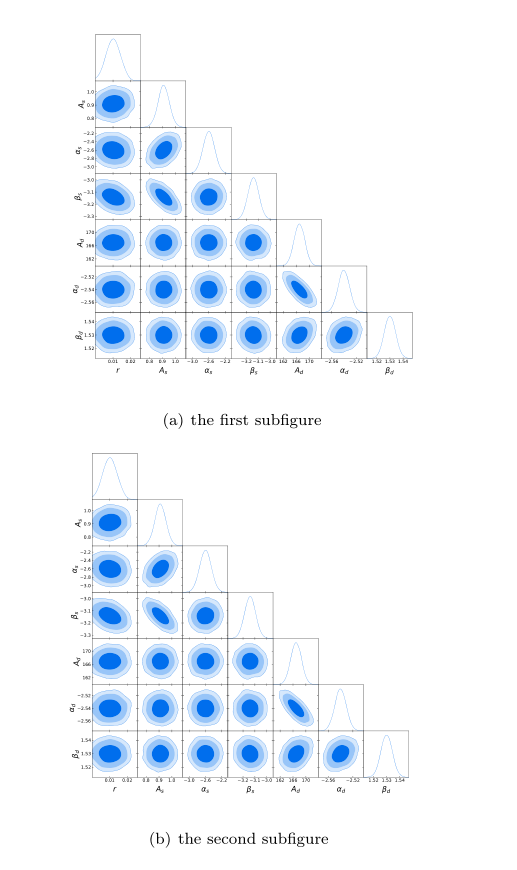只要将上面的方法使用//来换行即可，
\begin{figure}[htb]
\centering
\subfigure[the first subfigure]{
\begin{minipage}[c]{0.4\textwidth}
\includegraphics[width=1\textwidth]{41_95_220_large_corre2.eps} \\
\end{minipage}
}\\   #这是唯一不同的地方，增加了//
\subfigure[the second subfigure]{
\begin{minipage}[c]{0.4\textwidth}
\includegraphics[width=1\textwidth]{41_95_220_large_corre2.eps} \\
\end{minipage}
}
\end{figure}

如要修改图片大小，改变的是0.4的那个值，比如改为0.7
12.6 表格和表格并列
两个表格并列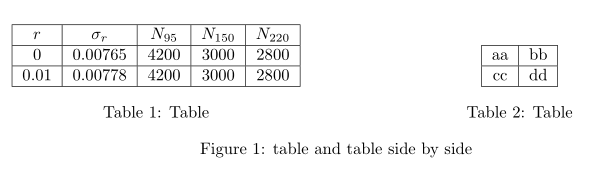\begin{figure}   %开始图片（表格和表格合成一个大的图片，这里指的是整体的大图片）
\begin{minipage}[b]{.5\linewidth}    %开辟第一个小页面，用来放第一个表格
\centering
\begin{tabular}{|c|c|c|c|c|}
\hline
$r$ & $\sigma_r$ & $N_{95}$ & $N_{150}$ & $N_{220}$\\
\hline
\multirow{1}*{0} & 0.00765 & 4200 & 3000 & 2800 \\
\hline
\multirow{1}*{0.01} & 0.00778 & 4200 & 3000 & 2800 \\
\hline
\end{tabular}
\captionof{table}{Table}
\end{minipage}
\begin{minipage}[b]{.5\linewidth}    %开辟第二个小页面，用来放表格
\centering
\begin{tabular}{|c|c|}
\hline
aa & bb \\ \hline
cc & dd \\ \hline
\end{tabular}
\captionof{table}{Table}
\end{minipage}
\caption{table and table side by side}
\end{figure}

可以看到有三个标注，可以通过加%去掉。另外，上面图中最后标注是figure 1 xxx, 然而我们是图表，所以只要将begin{figure}和end{figure}括号改为table即可，我就不再改了。
多个表格并列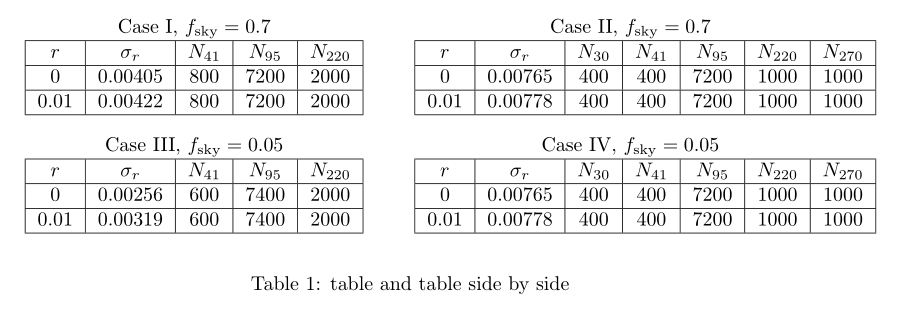\begin{table}   %开始图表
\begin{minipage}[b]{.5\linewidth}    %开辟第一个小页面，用来放第一个表格
\centering
Case I, $f_\text{sky}=0.7$
\begin{tabular}{|c|c|c|c|c|}
\hline
$r$ & $\sigma_r$ & $N_{41}$ & $N_{95}$ & $N_{220}$\\
\hline
\multirow{1}*{0} & 0.00405 & 800 & 7200 & 2000 \\
\hline
\multirow{1}*{0.01} & 0.00422 & 800 & 7200 & 2000 \\
\hline
\end{tabular}
\end{minipage}
\vspace{0.35cm}     %调节表格间距
\begin{minipage}[b]{.5\linewidth}    %开辟第二个小页面，用来放第二个表格
\centering
Case II, $f_\text{sky}=0.7$
\begin{tabular}{|c|c|c|c|c|c|c|}
\hline
%  \caption{The first table}
$r$ & $\sigma_r$ & $N_{30}$ & $N_{41}$ & $N_{95}$ & $N_{220}$ & $N_{270}$\\
\hline
\multirow{1}*{0} & 0.00765 & 400 & 400 & 7200 & 1000 & 1000 \\
\hline
\multirow{1}*{0.01} & 0.00778 & 400 & 400 & 7200 & 1000 & 1000 \\
\hline
\end{tabular}
\end{minipage}
\begin{minipage}[b]{.5\linewidth}    %开辟第三个小页面，用来放第三个表格
\centering
Case III, $f_\text{sky}=0.05$
\begin{tabular}{|c|c|c|c|c|}
\hline
$r$ & $\sigma_r$ & $N_{41}$ & $N_{95}$ & $N_{220}$\\
\hline
\multirow{1}*{0} & 0.00256 & 600 & 7400 & 2000 \\
\hline
\multirow{1}*{0.01} & 0.00319 & 600 & 7400 & 2000 \\
\hline
\end{tabular}
\end{minipage}
\vspace{0.35cm}
\begin{minipage}[b]{.5\linewidth}    %开辟第四个小页面，用来放第四个表格
\centering
Case IV, $f_\text{sky}=0.05$
\begin{tabular}{|c|c|c|c|c|c|c|}
\hline
%  \caption{The first table}
$r$ & $\sigma_r$ & $N_{30}$ & $N_{41}$ & $N_{95}$ & $N_{220}$ & $N_{270}$\\
\hline
\multirow{1}*{0} & 0.00765 & 400 & 400 & 7200 & 1000 & 1000 \\
\hline
\multirow{1}*{0.01} & 0.00778 & 400 & 400 & 7200 & 1000 & 1000 \\
\hline
\end{tabular}
\end{minipage}
\caption{table and table side by side}
\end{table}

注意，表格之间不要出现空行，因为空行表示换一段，所以表格会打乱。
12.7 更多关于表格的举例
这个内容可以参考Latex各种表格
举例1：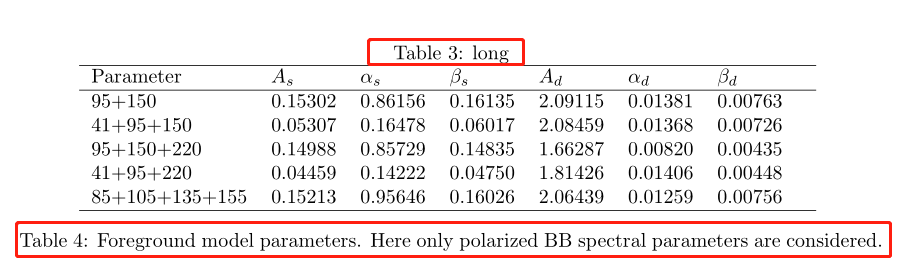先在开始加入两个包
\usepackage{xcolor}
\usepackage{colortbl,booktabs}

\begin{table}
\centering
\caption{long}
\begin{tabular*}{13cm}{lllllll}
\hline
Parameter & $A_s$ & $\alpha_s$ & $\beta_s$ & $A_d$& $\alpha_d$& $\beta_d$ \\
\hline
95+150 & 0.15302 & 0.86156 & 0.16135 & 2.09115 & 0.01381 & 0.00763\\
41+95+150 & 0.05307 & 0.16478 & 0.06017 & 2.08459 & 0.01368 & 0.00726\\
95+150+220 & 0.14988 & 0.85729 & 0.14835 & 1.66287 & 0.00820 & 0.00435\\
41+95+220 & 0.04459 & 0.14222 &  0.04750 & 1.81426 & 0.01406 & 0.00448\\
85+105+135+155 & 0.15213 & 0.95646 &  0.16026 & 2.06439 & 0.01259 & 0.00756\\
\hline
\end{tabular*}
\caption{Foreground model parameters. Here only polarized BB spectral parameters are considered.}\label{foreground parameters}
\end{table}

上面有两个caption可以选择去掉其一，其中{13cm}表示表格的长度是13cm，可以自行设定。
12.8 空行
空一行可以使用//来实现，但如果空了换行了还想继续空行使用//就会出错。此时使用\hspace*{\fill}\\
\hspace*{\fill}\\
\textbf{制图}

如下图，在“制图”前空了一行，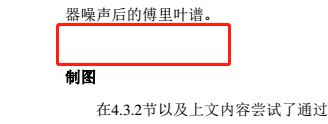13.交叉引用（corss-reference）
关于引用部分上面已经提及了一些，上面11的（4）我们用的是\ref {xxx}这个命令。这个命令有一个缺点是它只给出图、定理等的序号，比如“如图2所示”当我们使用\ref时需要写成：
如图\ref{xxx}所示

当我们并不知道这个\ref｛xxx｝是图还是表格还是定理等等的时候就比较麻烦，或者我们不想输入“图”，“表格”这样的字眼，这时可以使用宏包（同样，如果没有宏包先按照上面的方法安装）
\usepackage{hyperref}

然后将\ref {xxx}改为\autoref{xxx},e.g.
......
\usepackage{hyperref}
...
...
\begin{equation}
f(x)=
\left\{
\begin{array}{cc}
\int \mathrm{d}^3p\int_{0}^{\infty}\mathrm{d}x x^2 \frac{\delta(E-\sqrt{p^2+m^2})}{2E} , & x \geq 0 \\
0,& x < 0
\end{array}
\right.
\label{myequation}
\end{equation}
We all know \autoref{myequation} is a important equation

we can get: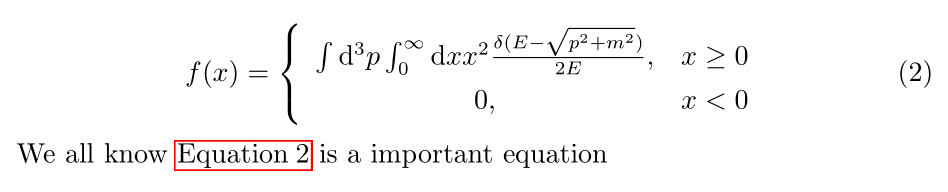可以看到自动加了equation这个词，而且是红色方框，点击可以直接跳到公式2.
14.文章布局
（1）页面大小
使用tex得到的pdf很窄，左右空的地方很大，如果我们想让它跟微软中的word一样的页面，可以在导言部分加入以下命令
\usepackage[top=2.54cm, bottom=2.54cm, left=3.18cm,right=3.18cm]{geometry}

（2）页眉页脚
参考文献见这里
使用宏包
\usepackage{fancyhdr}

在导言部分加入
\usepackage{fancyhdr}
\pagestyle{fancy}
\lhead{wudl}
\chead{$wudl's$ $tutorial article$}
\rhead{\thesection}
\cfoot{\thepage}

上面的结果是，页眉左上边是wudl，中间是chead中的句子，右上边是章节，页脚是页码。实际还有很多可选参数，自行查找需要的。
当然上面的页码是“1”“2”“3”这样的数，如果想要A of B,即第A页共B页，则要使用lastpage宏包。
如下：
\usepackage{fancyhdr}
\usepackage{lastpage}
\pagestyle{fancy}
\lhead{wudl}
\chead{$wudl's$ $tutorial article$}
\rhead{\thesection}
\cfoot{Page \thepage of \pageref{LastPage}}

结果为（3）列举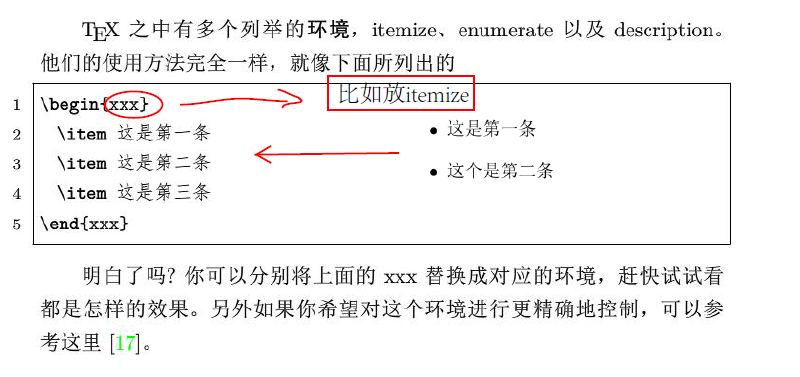（4）参考文献
A.手动输入
手动输入采用thebibliography环境，手动输入适合参考文献比较少的情况
  As is stated in \cite{bibitem1} blahblah\dots
\begin{thebibliography}{9}

\bibitem{bibitem1}
天朝. 朕的江山和子民[M]. 朕的天朝：天朝出版社. 2018

\bibitem{bibitem2}
blahblah(ditto)

这里\bibitem跟上面的列举中\item是一样的，上面的9表示最多可能有9条参考文献。结果为：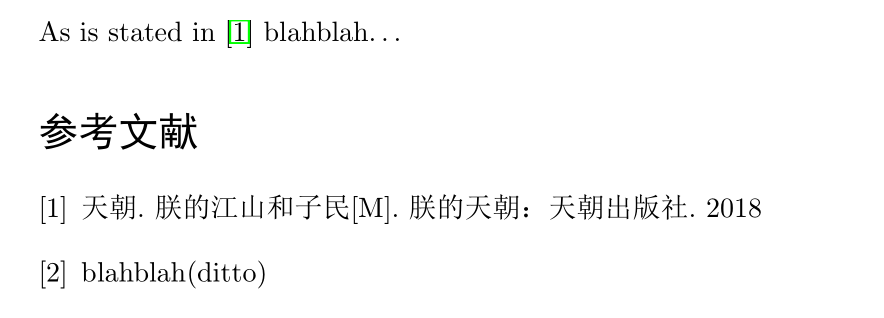B.使用Bibtex
这个比较复杂，以后再补充
15.超链接
使用
hyperref

实际上面都用过这个了。
16.插入代码
参考百度知道
%导言部分
...
...
\usepackage{listings}              %加入宏包
\lstset{language=Python}           %Python语言
\lstset{breaklines}                %自动将长的代码换行排版
\lstset{extendedchars=false}       %解决代码跨页时，章节标题，页眉等汉字不显示的问题
...
...
%需要插入代码的地方
\begin{lstlisting}
放入代码
\end{lstlisting}

这样的方法是代码是没有彩色的，结果如下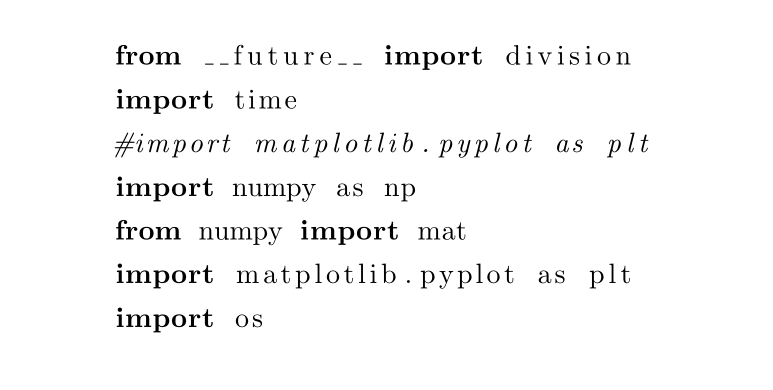解决彩色问题可以到网上找到已经修改彩色的代码，放入导言部分即可，实际上就是使用上文讲过的xcolor宏包自定义颜色。
例：自定义颜色（参考conding算子）
自定义颜色使用了listings和color两个宏包
\usepackage{listings}
\usepackage{color}

\definecolor{dkgreen}{rgb}{0,0.6,0}
\definecolor{gray}{rgb}{0.5,0.5,0.5}
\definecolor{mauve}{rgb}{0.58,0,0.82}

\lstset{ %
language=Octave,                % the language of the code
basicstyle=\footnotesize,           % the size of the fonts that are used for the code
numbers=left,                   % where to put the line-numbers
numberstyle=\tiny\color{gray},  % the style that is used for the line-numbers
stepnumber=2,                   % the step between two line-numbers. If it's 1, each line
% will be numbered
numbersep=5pt,                  % how far the line-numbers are from the code
backgroundcolor=\color{white},      % choose the background color. You must add \usepackage{color}
showspaces=false,               % show spaces adding particular underscores
showstringspaces=false,         % underline spaces within strings
showtabs=false,                 % show tabs within strings adding particular underscores
frame=single,                   % adds a frame around the code
rulecolor=\color{black},        % if not set, the frame-color may be changed on line-breaks within not-black text (e.g. commens (green here))
tabsize=2,                      % sets default tabsize to 2 spaces
captionpos=b,                   % sets the caption-position to bottom
breaklines=true,                % sets automatic line breaking
breakatwhitespace=false,        % sets if automatic breaks should only happen at whitespace
title=\lstname,                   % show the filename of files included with \lstinputlisting;
% also try caption instead of title
keywordstyle=\color{blue},          % keyword style
commentstyle=\color{dkgreen},       % comment style
stringstyle=\color{mauve},         % string literal style
escapeinside={\%*}{*)},            % if you want to add LaTeX within your code
morekeywords={*,...}               % if you want to add more keywords to the set
}

导入模块
参考gaojiaxing 和 instant以python为例，下载python版本的hightlighting,地址点这里。下载完成要将pythonhighlight.sty和放在工作目录下。
可以有两种方法来加载自己写的python代码。其一是将python代码在python自己的编辑器中写完保存为.py文件放到工作目录下（如test.py）,之后加载的时候只需要\inputpython…即可，这种方法可以节省大量的WinEdt书写空间
...
...
\usepackage{graphicx}
\usepackage{pythonhighlight}
...
...
\inputpython{test.py}{1}{41}#导入python文件test.py第一行到第41行
...

结果如下：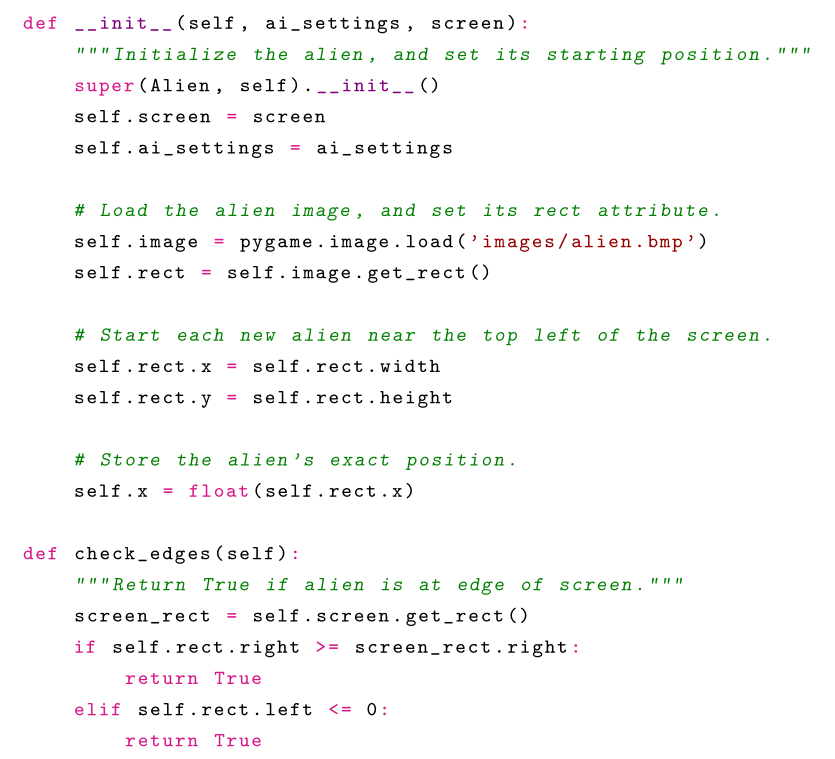其二，可以通过
\begin{xxx}
代码
\end{xxx}

例如：
  \begin{python}
#
from pyx import *
def f():
g = graph.graphxy(width=8)
g.plot(graph.data.function("y(x)=sin(x)/x", min=-15, max=15))
g.writePDFfile("function")
print r'\includegraphics{function}'
return f
\end{python}

结果如下；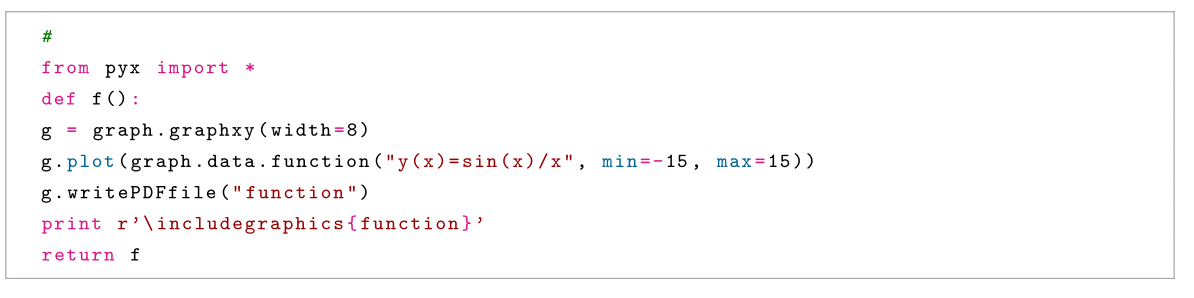17.目录生成
插入目录使用
\tableofcontents

注意这句话要在\begin{document}之后，end之前，例如：
\documentclass{article}
\usepackage{hyperref}
\begin{document}
\tableofcontents
\section{A section}
This is a section
\paragraph{A paragraph}
This is a paragraph
\subsection{A subsection}
This is a subsection
\end{document}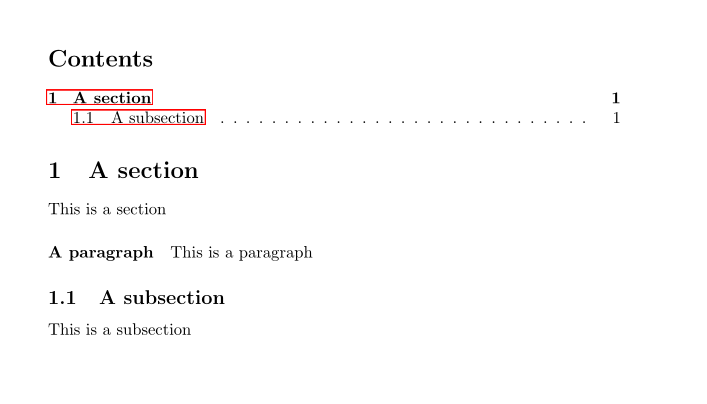注意上面的代码使用了超链接
\usepackage{hyerref}

如果没有这个超链接是不会有红色部分的。
18. 段落空行, 换行和首部缩进
在latex中，默认回车是不换行的，比如在latex中输入
A： Hello, how are you?
B： Hi, i'm fine.

结果为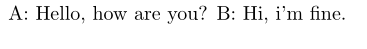我们实际想要的是B另起一行，此时可以通过加\来实现
A： Hello, how are you?\\
B： Hi, i'm fine.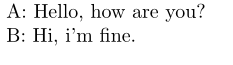另外，如果我们希望B换行之后行首缩进，比如写段落的时候希望前面缩进两个字符等，可以直接在latex中空一行
A： Hello, how are you?

B： Hi, i'm fine.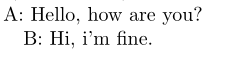最后，如果我们希望段落之间空的行数比较多，可以使用如下两种方法: 我在此就不翻译了，链接来自：
https://tex.stackexchange.com/questions/135134/how-to-add-an-empty-line-between-paragraphs
这里需要提到的是：在定义\newcommad的时候，可以将下面的\newcommand{…}…这几行代码放在\begin{document}之前或者之后，但是笔者习惯放之前，看着比较整洁易识别。另外就是newpara是自定义的名字，你可以把它定义成任何你想要的名字。最后，使用\newcommand的时候里面的\vskip 1cm默认单位是cm，而不是inch，而第1种方法是inch.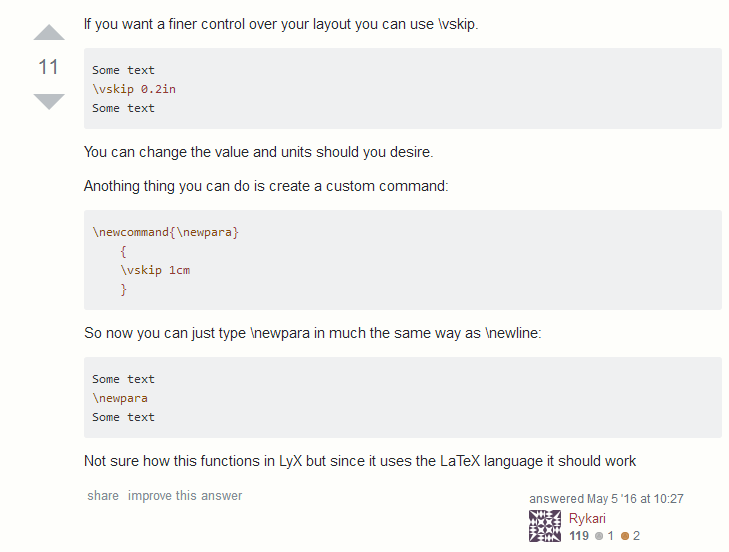展开全文• http://blog.sina.com.cn/s/blog_6da2b94d0101qspb.htmlhttps://jingyan.baidu.com/article/7e44095335ff172fc1e2ef11.html
http://blog.sina.com.cn/s/blog_6da2b94d0101qspb.htmlhttps://jingyan.baidu.com/article/7e44095335ff172fc1e2ef11.html
展开全文• 这不是最后一篇，明天开始建模所以会从6号开始继续更新。前情回顾【LaTeX】E喵的...【LaTeX】E喵的LaTeX新手入门教程（4）图表参考文献天下文章一大抄，抄来抄去有提高。 ——白岩松常备工具：JabRef>>戳我下载JabRef

这不是最后一篇，明天开始建模所以会从6号开始继续更新。前情回顾【LaTeX】E喵的LaTeX新手入门教程（1）准备篇 【LaTeX】E喵的LaTeX新手入门教程（2）基础排版 【LaTeX】E喵的LaTeX新手入门教程（3）数学公式 【LaTeX】E喵的LaTeX新手入门教程（4）图表参考文献天下文章一大抄，抄来抄去有提高。
——白岩松常备工具：JabRef>>戳我下载<<JabRef是一款管理参考文献用的软件，相当好用。装好了以后在选项中把这两个选项改成如图示的样子。*nix用户可以尝试用用KBibTeX这个来自于KDE的软件，自动搜索什么的真是炫酷。JabRef使用的参考文献数据库是.bib格式的，这也正是LaTeX参考文献方案BibTeX所使用的文件，果断是绝配。由于一些奇怪的原因，JabRef中输入的文献名称和属性中，中文都是小框框。→_→大家摸着黑打吧。使用JabRef创建参考文献数据库打开JabRef，选择『文件』→『新建数据库』就完成了0w0现在我们来添加要引用的文献。点击工具栏上的『+』号：然后选择记录类型，也就是你要引用的文章类型，是论文还是其他的什么的。这里我们选个Article作为示例。这时就会有编辑框，把该填的地方都填了就可以了。我们且胡乱填一个。其中，BibTeXKey是我们在文档中引用该文献需要的东西，记不住了到这查一下就可以了。然后把它保存到和你的.tex源文件的相同目录下。插入参考文献我们有了.bib文件之后，就可以在LaTeX文档中插入参考文献了。要想在正文某处引用插入的参考文献，使用\cite{BibTeXKey}来引用。其中的BibTeXKey就是我们在JabRef中填写的那个BibTeXKey。同时，我们也需要在文档的最后建立一个参考文献列表。建立参考文献列表的方法极其简单：先指定参考文献列表的格式，用\bibliographystyle加一个格式参数。LaTeX内置的格式共有以下几种：
plain，按字母的顺序排列，比较次序为作者、年度和标题.unsrt，样式同plain，只是按照引用的先后排序.alpha，用作者名首字母+年份后两位作标号，以字母顺序排序.abbrv，类似plain，将月份全拼改为缩写，更显紧凑.ieeetr，国际电气电子工程师协会期刊样式.acm，美国计算机学会期刊样式.siam，美国工业和应用数学学会期刊样式.apalike，美国心理学学会期刊样式.
除了以上的内置格式之外，也可以自己编写一个.bst文件来描述参考文献格式，这时\bibliographystyle的参数写你的bst文件不带扩展名的文件名就可以了。当然这个文件的语法很坑爹，所以一般情况下就用别人写好的就好。。。指定完了格式，在后面加上一个\bibliography{参考文献数据库文件名不带扩展名的}，就算大功告成。说了这么多，不如动手实践一下我们就拿刚才的参考文献数据库来用一下。（不要纠结中文，这只是个示例，你可以打英文→_→）自古以来，喵星人的文明程度就超前于人类数千年之久。
因此，学习喵星人的先进文明，对于人类的发展有着巨大的好处。
喵星人的先进文明包括科技与文化两个方面\cite{mxr2013}。
\bibliographystyle{plain}
\bibliography{reference}编译带有参考文献的文档的流程比较奇怪，大家跟我来：
xelatex编译一遍bibtex编译一遍xelatex编译一遍xelatex编译一遍
以上编译的都是你的.tex文件。BibTeX的编译按钮大家的工具栏上都有的。编译好后就可以看看效果啦~将『参考文献』插入目录这时如果我们使用\tableofcontents生成一个目录的话，是看不到参考文献的目录项的。要想将它加入，就必须手动加入。
在\bibliography后面加上一句就可以了\addcontentsline{toc}{级别}{目录项名称}级别可以填写文档结构的级别，如section、chapter等。再来个标题！这东西本来应该在第二期说的，当时给忘了。\title{标题}
\author{作者}
\date{日期}
\maketitle其中前三行可以写在导言区。最终成品的帅气文档！再次提示：这里出现了中文是因为本喵在导言区里加了其他的东西，乃们在实际编译时会看不到中文，此示例代码仅供娱乐。\title{向喵星人学习}
\author{E.~Meow}
\date{\today}
\maketitle
\tableofcontents
\section{引论}
自古以来，喵星人的文明程度就超前于人类数千年之久。
因此，学习喵星人的先进文明，对于人类的发展有着巨大的好处。
喵星人的先进文明包括科技与文化两个方面\cite{mxr2013}。
\bibliographystyle{plain}
\bibliography{reference}
\addcontentsline{toc}{section}{References}大型文章的组织LaTeX在编译文档时，内部申请的内存资源是有限的，对于太长的文档有时可能会编译失败，因此把文章的各部分单独分出来，编译器省心，看着也觉得整齐。对于大型文档，一般进行这样的拆分：
一个主文档，包括你所有的导言区内容，正文使用\include包含文档各部分，最后加上参考文献那三句。若干个文档部分，不用写导言区也不用写\begin{document}和\end{document}，只写文档正文。
还是拿刚才的成型文档示例。% This is main.tex
\documentclass{article}
% ===== 中文支持部分，请无视 =====
\usepackage{xeCJK}
\setCJKmainfont[BoldFont={黑体}]{宋体}
% ===== 结束 =====
\title{向喵星人学习}
\author{E.~Meow}
\date{\today}
\begin{document}
\maketitle
\tableofcontents
\include{intro}
\bibliographystyle{plain}
\bibliography{reference}
\addcontentsline{toc}{section}{References}
\end{document}-% This is intro.tex
\section{引论}
自古以来，喵星人的文明程度就超前于人类数千年之久。
因此，学习喵星人的先进文明，对于人类的发展有着巨大的好处。
喵星人的先进文明包括科技与文化两个方面\cite{mxr2013}。编译的时候仅编译main.tex即可，编译效果见前面的图，一样的。下期预告中文！from: http://blog.renren.com/blog/339175058/894159975?bfrom=01020110200

展开全文新手 入门
• 原文地址：傻瓜版Latex（WinEdt）的bib参考文献管理教程，3分钟上手作者：brekeley 写了几篇paper，都是采用别人给的模板，里面的参考文献都是在最后手动敲出来的，比较麻烦，而且特别容易出错。这短时间就深刻体会...java python github 大数据 数据库
• LaTeX期刊论文模板教程-以Physical Review系列为例写在前面在准备给期刊投稿的时候，用LaTeX写对应期刊格式的论文就成了必不可少的一个重要工作。每个期刊都有着不同的格式，因此学会如何应用这样的论文格式模板便是...
• 今天要使用latex写report,需要加入引用和参考文献,在网上查了很多都让人有些迷惑,最后终于成功,并写下本博客为方便其他人使用. 流程: 1.创建bib文件,这其实就是一个文本文件,以.bib后缀结尾. 2.在bib文件里添加...
• Latex参考文献引用问题BibTex的使用 最近因为在写论文，考虑用Latex排版，之前没用过Latex，算是新手小白，第一次用花了很多时间，记录一下参考文献相关的问题。 BibTex的使用 这一部分的使用，网上已经有很多教程了...
• 如何使用latex引用参考文献可以参考我之前的一篇博客：latex参考文献引用【bibtex】 仍然使用的是IEEEtrans的参考文献格式，但是，我像网上的教程，使用hyperref的时候，发现无法正常进行链接。百度了好多，都是前...hyperref cite 链接
• 根据官网教程实现 1、创建一个Latex文件，命名随意，如 myarticle.tex \documentclass[11pt]{article} \usepackage{cite} \begin{document} \title{My Article} \author{Nobody Jr.} \date{Today} \maketitle ...
• 文章目录Latex排版教程1. Latex源文件的基本结构2. 大纲目录3. 文字设置3.1字体族设置3.1.1英文3.1.2中文3.2字体粗细宽度设置3.3 字体形状设置3.4字体大小...参考文献 Latex排版教程 教程来自b站–latex中文教程-15集
• 参考：https://blog.csdn.net/zaishuiyifangxym/article/details/88170827 ... 解决文献不显示 按照其他的教程操作 然后在工具中，选择F6 然后再F8,然后再F6 ...
• LATEX教程第五讲——让Latex富有层次5.1 标题、作者和日期5.2 摘要和关键词5.3 将文章分层5.4 目录5.5 参考文献5.6 附录5.7 章节不编号 这一讲主要来说一些Latex中分节分段的问题，如果需要宏包还请参考第二讲到第四...
• 最近在改一篇论文的时候，发现一件很奇怪的事情，一个引用我已经删除了，但是在文末的参考文献依旧存在。 问题分析 正常来说使用bib文件来引用参考文献，如果没有引用的话，是不会显示的，如果需要全部显示需要...
• 由于Zotero更新到了5.0，我的...这篇教程正是来帮助大家管理参考文献的。无论你是想撰写docx格式或者LaTeX格式的论文，跟随这篇教程的脚步，将会省下不少时间。 一、需要准备的软件 这次要给大家推荐的神器叫做Zo...Zotero
• 转载 LaTeX新人教程，一小时从完全陌生到基本入门 作者 : 董楠 LaTeX新人教程，一小时从完全陌生到基本入门 ...后来应小伙伴呼声，加入了图片引用、公式引用和参考文献内容。 因此如果要走完这个流...
• 转载 LaTeX新人教程，一小时从完全陌生到基本入门 作者 : 董楠LaTeX新人教程，一小时从完全陌生到基本入门by Nan 对于真心渴望迅速上手...后来应小伙伴呼声，加入了图片引用、公式引用和参考文献内容。 因此如果要走
• 转载自一篇实用的Latex的入门教程转载自作者 : 董楠 LaTeX新人教程，一小时从完全陌生到基本入门 by Nan对于真心渴望迅速...后来应小伙伴呼声，加入了图片引用、公式引用和参考文献内容。 因此如果要走完这个流程
• 本文是一篇适合排版参考文献较多的论文的教程，目的如题。 在文献管理工具中将题录导出为BibTex 打开这个文件，尽管是.txt结尾，内部格式应当是这样的 注意红框的内容，一会要用到。 在TexStudio里面新建一个...
• 参考文献[1, 3] 对LATEX 系统提供了完整的描述。 对大多数计算机，从个人计算机（PC）和Mac 到大型的UNIX 和 VMS 系统，LATEX 都有适用版本。在许多大学的计算机机房，你会发现系 统中已经安装了LATEX 并能够使用。...
• LATEX教程第三讲—— 那些不得不说的宏包（中）3.1 表格与列表arraylongtablesupertabulartabularxmultirowhhlineslashboxbooktabs3.2 目录与索引toclofttitletocmultitocminitoctocbibindshorttoc3.3 参考文献...
• 之前用LaTeX写论文时，参考文献都是手动添加管理的，真是让人很抓狂。所以这次趁着假期，简单看了一下怎么使用BibTeX对参考文献进行管理，这里以IEEE的最新模板为例。 首先说明，我之前用的是MiKTex和TeXnicCenter...
• LaTeX + sublime Text + Sumatra PDF反向搜索 + bib格式文件文献引用（及国标化配置） 找了一大堆教程没有一个把细节都一篇文章讲完的。于是自己写一篇 安装TEx live 2017和Sumatra PDF： 地址：...
• 安装LateX中文教程参考文献】 TeXLive安装 1.下载 TeXLive通过官网下载会比较慢，这里推荐通过清华大学开源软件镜像站进行下载，下载链接：https://mirrors.tuna.tsinghua.edu.cn/ctan/systems/tex...windows
• 文章目录摘要第一个文档标题、作者和注释章节和段落加入目录换行数学公式插入图片简单表格结尾中文支持宏包模板参考文献和制作幻灯片致谢 这事一篇老帖子，今天把它翻出来是因为这个帖子对于LaTeX入门来说确实非常...markdown ieee论文
• LaTeX 文档排版教程 第一部分：从零开始 第一节：开始使用 第二节：快速入门 第三节：撰写文本 第四节：撰写公式 第五节：错误处理 ...第三节：参考文献 第四节：全文索引 第四部分：版面设...